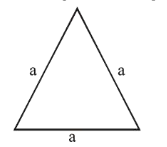# A copper wire is wound on a wooden frame,

Question:

A copper wire is wound on a wooden frame, whose shape is that of an equilateral triangle. If the linear dimension of each side of the frame is increased by a factor of 3 , keeping the number of turns of the coil per unit length of the frame the same, then the self inductance of the coil:

1. (1) decreases by a factor of 9

2. (2) increases by a factor of 27

3. (3) increases by a factor of 3

4. (4) decreases by a factor of $9 \sqrt{3}$

Correct Option: , 3

Solution:

(3) As total length $\mathrm{L}$ of the wire will remain constant

$\mathrm{L}=(3 \mathrm{a}) \mathrm{N}$      $(\mathrm{N}=$ total turns $)$

and length of winding $=($ d $) \mathrm{N}$$(\mathrm{d}=$ diameter of wire $)$

self inductance $=\mu_{0} \mathrm{n}^{2} \mathrm{~A} \ell$

$=\mu_{0} n^{2}\left(\frac{\sqrt{3} a^{2}}{4}\right) d N$

$\propto \mathrm{a}^{2} \mathrm{~N} \propto \mathrm{a}\left[\mathrm{as} \mathrm{N}=\mathrm{L} / 3 \mathrm{a} \Rightarrow \mathrm{N} \propto \frac{1}{\mathrm{a}}\right]$

Now 'a' increased to ' $3 a$ '

So self inductance will become 3 times